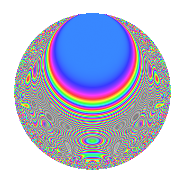# Properties

 Label 403.2.sLevel 403 Weight 2 Character orbit s Rep. character $$\chi_{403}(160,\cdot)$$ Character field $$\Q(\zeta_{6})$$ Dimension 70 Newforms 1 Sturm bound 74 Trace bound 0

# Related objects

## Defining parameters

 Level: $$N$$ = $$403 = 13 \cdot 31$$ Weight: $$k$$ = $$2$$ Character orbit: $$[\chi]$$ = 403.s (of order $$6$$ and degree $$2$$) Character conductor: $$\operatorname{cond}(\chi)$$ = $$403$$ Character field: $$\Q(\zeta_{6})$$ Newforms: $$1$$ Sturm bound: $$74$$ Trace bound: $$0$$

## Dimensions

The following table gives the dimensions of various subspaces of $$M_{2}(403, [\chi])$$.

Total New Old
Modular forms 78 78 0
Cusp forms 70 70 0
Eisenstein series 8 8 0

## Trace form

 $$70q - 6q^{2} - 2q^{3} + 30q^{4} - 29q^{9} + O(q^{10})$$ $$70q - 6q^{2} - 2q^{3} + 30q^{4} - 29q^{9} + 2q^{10} + 13q^{12} + q^{13} - 14q^{14} - 15q^{15} - 28q^{16} - 12q^{17} - 3q^{20} - 9q^{21} + 4q^{22} + 10q^{23} + 18q^{24} + 19q^{25} + 6q^{26} + 34q^{27} - 33q^{28} - 18q^{29} - 31q^{30} - 2q^{31} + 36q^{32} - 12q^{33} + 9q^{34} - 12q^{35} - 16q^{36} - 18q^{37} - 21q^{38} - 30q^{39} + 5q^{40} + 98q^{42} - 38q^{43} + 42q^{44} - 6q^{46} + 54q^{48} - 18q^{49} - 51q^{50} - 7q^{51} + 41q^{52} - 22q^{53} + 18q^{54} - 15q^{55} - 50q^{56} + 15q^{57} - 12q^{58} - 13q^{61} - 23q^{62} - 6q^{63} - 38q^{64} - 12q^{65} - 52q^{66} - 44q^{68} + 32q^{69} + 27q^{71} - 15q^{72} - 9q^{73} + 38q^{74} - 50q^{75} + 126q^{76} + 34q^{77} + 14q^{78} + 6q^{79} - 11q^{81} + 39q^{82} - 54q^{83} + 15q^{84} - 33q^{85} - 24q^{86} + 28q^{87} - 32q^{88} - 6q^{89} - 11q^{90} - 70q^{91} - 6q^{92} + 14q^{93} - 43q^{94} + 25q^{95} + 36q^{96} - 75q^{97} + 93q^{99} + O(q^{100})$$

## Decomposition of $$S_{2}^{\mathrm{new}}(403, [\chi])$$ into irreducible Hecke orbits

Label Dim. $$A$$ Field CM Traces $q$-expansion
$$a_2$$ $$a_3$$ $$a_5$$ $$a_7$$
403.2.s.a $$70$$ $$3.218$$ None $$-6$$ $$-2$$ $$0$$ $$0$$